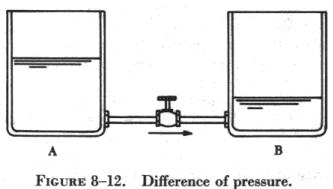ELECTROMOTIVEFORCE ELECTROMOTIVE FORCE

The flow of electrons from a negative point to a positive point is called an electric current; this current flows because of a difference in electric pressure between the two points.

If an excess of electrons with a negative charge exists at one end of a conductor and a deficiency of electrons with a positive charge at the other, an electrostatic field exists between the two charges. Electrons are repelled from the negatively charged point and are attracted by the positively charged point.The flow of electrons of electric current can be compared to the flow of water between two interconnected water tanks when a difference of pressure exists between two tanks. Figure 8-12 shows the level of water in tank A to be at a higher level than the water level in tank B. If the valve in the interconnecting line between the tanks is opened, water will flow from tank A into tank B until the level of water is the same in both tanks.

It is important to note that it was not the pressure in tank A that caused the water to flow; rather, it was the difference in pressure between tank A and tank B that caused the flow. When the water in the two tanks are at the same level, the flow of water ceases because there is no longer a difference of pressure.

This comparison illustrates the principle that causes the electrons to move, when a proper path is available, from a point of excess electrons to a point deficient in electrons. The force that causes this movement is the potential difference in electrical energy between the two points. This force is called the electrical pressure or the potential difference or the electromotive force (electron moving force) which can all be considered the same thing. Electromotive force, abbreviated e.m.f., causes current (electrons) to move in an electric path or circuit. The practical unit of measurement of e.m.f., or potential difference, is the volt. The symbol for e.m.f. is the capital letter "E."

If the water pressure in tank A of figure 8-12 is 10 psi and the pressure in tank B is 2 psi, there is a difference in pressure of 8 psi. Similarly, it can be said that an electromotive force of 8 volts exists between two electrical points. Since potential difference is measured in volts, the word "voltage" can also be used to describe amounts of potential difference. Thus, it is correct to say that the voltage of a certain aircraft battery is 24 volts, another means of indicating that a potential difference of 24 volts exists between two points connected by a conductor.